### THE ALGEBRA OF SUMMATION NOTATION

The following problems involve the algebra (manipulation) of summation notation. Summation notation is used to define the definite integral of a continuous function of one variable on a closed interval. Let's first briefly define summation notation. If f(i) represents some expression (function) involving i, then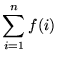has the following meaning :.

The "i=" part underneath the summation sign tells you which number to first plug into the given expression. The number on top of the summation sign tells you the last number to plug into the given expression. You always increase by one at each successive step. For example,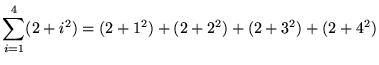= 3 + 6 + 11 + 18

= 38 .

We will need the following well-known summation rules.

1.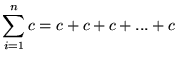(n times) = cn, where c is a constant.
2..
3.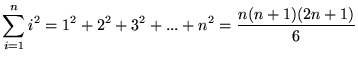.
4..

Most of the following problems are average. A few are somewhat challenging. If you are going to try these problems before looking at the solutions, you can avoid common mistakes by using the formulas given above in exactly the form that they are given. For instance, make sure that a summation begins with i=1 before using the above formulas.
• PROBLEM 1 : Evaluate.

• PROBLEM 2 : Evaluate.

• PROBLEM 3 : Evaluate.

• PROBLEM 4 : Evaluate.

• PROBLEM 5 : Evaluate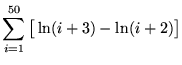.

• PROBLEM 6 : Evaluate.

• PROBLEM 7 : Evaluate.

• PROBLEM 8 : Evaluate.

• PROBLEM 9 : Evaluate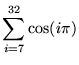.

• PROBLEM 10 : Prove that.

• PROBLEM 11 : Prove that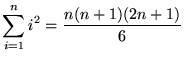.

• PROBLEM 12 : Evaluate.

• PROBLEM 13 : Evaluate.

• PROBLEM 14 : Compute the sum of the first 120 numbers in the following list : 3, 7, 11, 15, 19, 23, 27, ... .